# Transfer Function of Armature Controlled DC Motor

0
950

## Transfer Function of Armature Controlled DC Motor:

#### The electrical system consists of the armature and the field circuit but for analysis purpose, only the armature circuit is considered because the field is excited by a constant voltage.The mechanical system consist of the rotating part of the motor and load connected to the shaft of the motor.The armature controlled DC motor speed control system is shown in the below figure.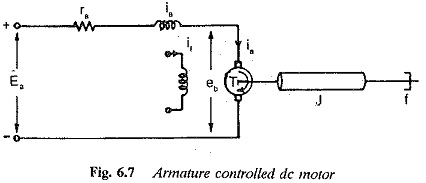#### The equivalent circuit of armature is shown in the below figure.#### By Kirchoff’s voltage law, we can write,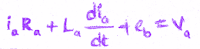#### Torque of DC motor is proportional to the product of flux and current.Since flux is constant in this system, the torque is proportional to ia alone.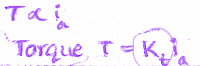#### The mechanical system of the motor is shown in the below figure.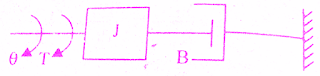#### The back emf of DC machine is proportional to speed (angular velocity) of shaft.#### The differential equations governing the armature controlled DC motor speed control system are#### On equating the above equations (2),(3) we get=> (5)

#### Substituting for Eb(s) & Ia(s) from (4),(5) respectively in equation (6)#### The required transfer function of armature controlled dc motor  is θ(s)/Va(s)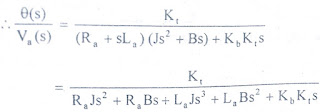=> (7)#### The transfer function of armature controlled dc motor can be expressed in another standard form as shown below.From the equation (7) we get,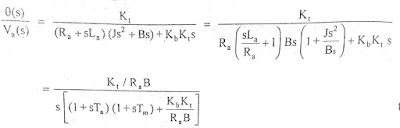#### J/B = Tm = Mechanical time constant

READ HERE  Swinburne Test of DC Machine